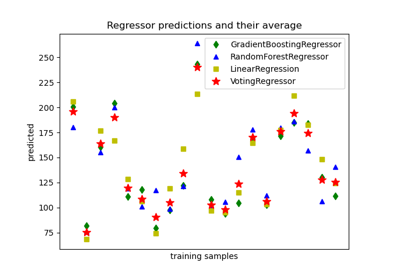# sklearn.ensemble.VotingRegressor¶

class sklearn.ensemble.VotingRegressor(estimators, *, weights=None, n_jobs=None, verbose=False)[source]

Prediction voting regressor for unfitted estimators.

New in version 0.21.

A voting regressor is an ensemble meta-estimator that fits several base regressors, each on the whole dataset. Then it averages the individual predictions to form a final prediction.

Read more in the User Guide.

Parameters
estimatorslist of (str, estimator) tuples

Invoking the fit method on the VotingRegressor will fit clones of those original estimators that will be stored in the class attribute self.estimators_. An estimator can be set to 'drop' using set_params.

Changed in version 0.21: 'drop' is accepted.

Deprecated since version 0.22: Using None to drop an estimator is deprecated in 0.22 and support will be dropped in 0.24. Use the string 'drop' instead.

weightsarray-like of shape (n_regressors,), default=None

Sequence of weights (float or int) to weight the occurrences of predicted values before averaging. Uses uniform weights if None.

n_jobsint, default=None

The number of jobs to run in parallel for fit. None means 1 unless in a joblib.parallel_backend context. -1 means using all processors. See Glossary for more details.

verbosebool, default=False

If True, the time elapsed while fitting will be printed as it is completed.

Attributes
estimators_list of regressors

The collection of fitted sub-estimators as defined in estimators that are not ‘drop’.

named_estimators_Bunch

Attribute to access any fitted sub-estimators by name.

New in version 0.20.

VotingClassifier

Soft Voting/Majority Rule classifier.

Examples

>>> import numpy as np
>>> from sklearn.linear_model import LinearRegression
>>> from sklearn.ensemble import RandomForestRegressor
>>> from sklearn.ensemble import VotingRegressor
>>> r1 = LinearRegression()
>>> r2 = RandomForestRegressor(n_estimators=10, random_state=1)
>>> X = np.array([[1, 1], [2, 4], [3, 9], [4, 16], [5, 25], [6, 36]])
>>> y = np.array([2, 6, 12, 20, 30, 42])
>>> er = VotingRegressor([('lr', r1), ('rf', r2)])
>>> print(er.fit(X, y).predict(X))
[ 3.3  5.7 11.8 19.7 28.  40.3]


Methods

 fit(X, y[, sample_weight]) Fit the estimators. fit_transform(X[, y]) Fit to data, then transform it. get_params([deep]) Get the parameters of an estimator from the ensemble. Predict regression target for X. score(X, y[, sample_weight]) Return the coefficient of determination R^2 of the prediction. set_params(**params) Set the parameters of an estimator from the ensemble. Return predictions for X for each estimator.
__init__(estimators, *, weights=None, n_jobs=None, verbose=False)[source]

Initialize self. See help(type(self)) for accurate signature.

fit(X, y, sample_weight=None)[source]

Fit the estimators.

Parameters
X{array-like, sparse matrix} of shape (n_samples, n_features)

Training vectors, where n_samples is the number of samples and n_features is the number of features.

yarray-like of shape (n_samples,)

Target values.

sample_weightarray-like of shape (n_samples,), default=None

Sample weights. If None, then samples are equally weighted. Note that this is supported only if all underlying estimators support sample weights.

Returns
selfobject

Fitted estimator.

fit_transform(X, y=None, **fit_params)[source]

Fit to data, then transform it.

Fits transformer to X and y with optional parameters fit_params and returns a transformed version of X.

Parameters
X{array-like, sparse matrix, dataframe} of shape (n_samples, n_features)
yndarray of shape (n_samples,), default=None

Target values.

**fit_paramsdict

Returns
X_newndarray array of shape (n_samples, n_features_new)

Transformed array.

get_params(deep=True)[source]

Get the parameters of an estimator from the ensemble.

Parameters
deepbool, default=True

Setting it to True gets the various classifiers and the parameters of the classifiers as well.

predict(X)[source]

Predict regression target for X.

The predicted regression target of an input sample is computed as the mean predicted regression targets of the estimators in the ensemble.

Parameters
X{array-like, sparse matrix} of shape (n_samples, n_features)

The input samples.

Returns
yndarray of shape (n_samples,)

The predicted values.

score(X, y, sample_weight=None)[source]

Return the coefficient of determination R^2 of the prediction.

The coefficient R^2 is defined as (1 - u/v), where u is the residual sum of squares ((y_true - y_pred) ** 2).sum() and v is the total sum of squares ((y_true - y_true.mean()) ** 2).sum(). The best possible score is 1.0 and it can be negative (because the model can be arbitrarily worse). A constant model that always predicts the expected value of y, disregarding the input features, would get a R^2 score of 0.0.

Parameters
Xarray-like of shape (n_samples, n_features)

Test samples. For some estimators this may be a precomputed kernel matrix or a list of generic objects instead, shape = (n_samples, n_samples_fitted), where n_samples_fitted is the number of samples used in the fitting for the estimator.

yarray-like of shape (n_samples,) or (n_samples, n_outputs)

True values for X.

sample_weightarray-like of shape (n_samples,), default=None

Sample weights.

Returns
scorefloat

R^2 of self.predict(X) wrt. y.

Notes

The R2 score used when calling score on a regressor uses multioutput='uniform_average' from version 0.23 to keep consistent with default value of r2_score. This influences the score method of all the multioutput regressors (except for MultiOutputRegressor).

set_params(**params)[source]

Set the parameters of an estimator from the ensemble.

Valid parameter keys can be listed with get_params().

Parameters
**paramskeyword arguments

Specific parameters using e.g. set_params(parameter_name=new_value). In addition, to setting the parameters of the stacking estimator, the individual estimator of the stacking estimators can also be set, or can be removed by setting them to ‘drop’.

transform(X)[source]

Return predictions for X for each estimator.

Parameters
X{array-like, sparse matrix} of shape (n_samples, n_features)

The input samples.

Returns
predictions: ndarray of shape (n_samples, n_classifiers)

Values predicted by each regressor.

## Examples using sklearn.ensemble.VotingRegressor¶